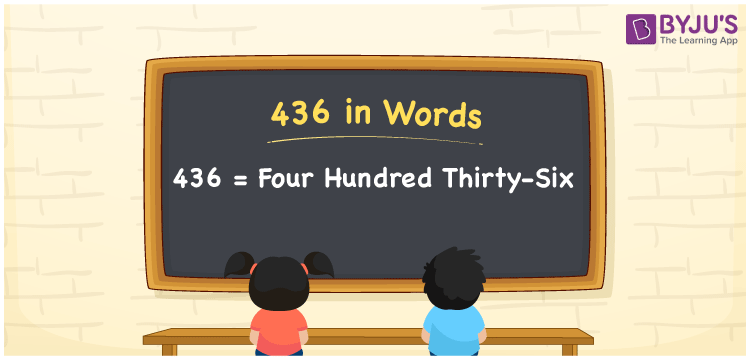# 436 in Words

436 in words is written as Four hundred thirty-six. In both the International System of Numerals and the Indian System of Numerals, 436 is written as Four hundred thirty-six. The number 436 is a Cardinal Number as it represents some quantity. For example, the “cost of the t-shirt is 436 rupees”.

 436 in Words Four hundred thirty-six Four hundred thirty-six in Number 436

## 436 in English Words

We write 436 in English Words using the letters of the English alphabet. Therefore, we read 436 in English as “Four hundred thirty-six”.## How to Write 436 in Words?

To write 436 in words, we shall use the place value chart. In the place value chart, write 4 in the hundreds, 3 in the tens, and 6 in the ones, respectively. Now let us make a place value chart to write the number 436 in words.

 Hundreds Tens Ones 4 3 6

Thus, we can write the expanded form as

4 × Hundred + 3 × Ten + 6 × One

= 4 × 100 + 3 × 10 + 6 × 1

= 400 + 30 + 6

= 436

= Four hundred thirty-six.

436 is a natural number, the successor of 435 and the predecessor of 437.

436 in words – Four hundred thirty-six

• Is 436 an odd number? – No
• Is 436 an even number? – Yes
• Is 436 a perfect square number? – No
• Is 436 a perfect cube number? – No
• Is 436 a prime number? – No
• Is 436 a composite number? – Yes

## Frequently Asked Questions on 436 in Words

Q1

### How to write 436 in words?

436 in words is written as Four hundred thirty-six.
Q2

### How to write 436 in the International and Indian System of Numerals?

In both, the system of numerals, 436 in words, is written as Four hundred thirty-six.
Q3

### What is the successor of 436?

The successor of 436 is 437.